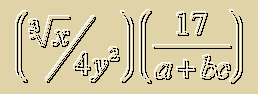# Expression EvaluationUnderstanding mathematics notation is, well, essential to understanding mathematics. The material here discusses what we mean by the concept of an expression, and how one reads an expression in order to evaluate it. You evaluate an expression in order to find its value. The expression's value is what number it equals.

We will center on the concepts of operators and operands and look at expressions as if they were animated in our imagination. A few animations are presented in an effort to get these ideas across.

Precedence is covered here, and usually students learn that quite early in their study of mathematics. Often, though, a person is not exposed to the following way of interpreting a math expression in terms of its operators and operands.